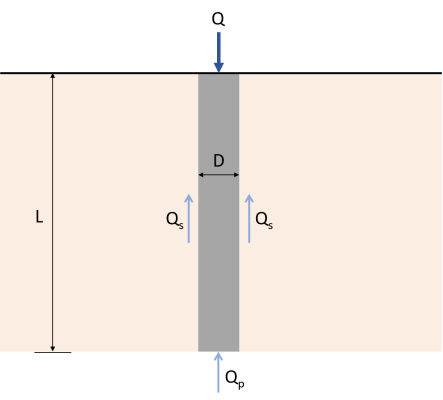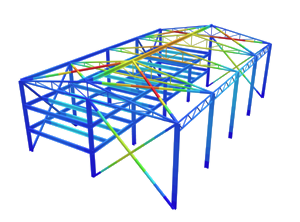SkyCiv Documentation

Your guide to SkyCiv software - tutorials, how-to guides and technical articles

1. Home
2. SkyCiv Foundation
3. Piles
4. Geotechnical Capacity of Piles

# Geotechnical Capacity of Piles

## How to calculate the ultimate load-carrying capacity of a single pile with examples

Evaluating the ultimate load-carrying capacity of a single pile is an important aspect of pile design that can sometimes be complicated. To easily understand the load transfer mechanism of a single pile, imagine a concrete pile of length L with diameter D, as shown in Figure 1.Figure 1: Load transfer Mechanism for piles

The load Q applied on the pile shall be transferred directly to the soil. Part of this load will be resisted by the side or skin friction developed along the shaft (Qs) and the rest will be resisted by the soil below the tip of the pile (Qp).  Therefore, the ultimate load-carrying capacity (Qu) of a pile shall be given by the equation (1).  Numerous studies and methods are available to estimate the values of Qp and Qs.

$${Q}_{u} = {Q}_{p} + {Q}_{s}$$    (1)

Qs = Skin-frictional resistance

Want to try SkyCiv’s Foundation Design software? Our free tool allows users to perform load-carrying calculations without any download or installation!

## End-bearing Capacity

Ultimate end-bearing capacity is theoretically the maximum load per unit area which can be supported by the soil without failure. The equation of Karl Von Terzaghi, father of soil mechanics, is one of the first and most used theory on evaluating the ultimate bearing capacity of foundations. Terzaghi’s equation for ultimate bearing capacity can be expressed as:

$${q}_{u} = (c × {N}_{c}) + (q × {N}_{q}) + (\frac{1}{2} × γ × B × {N}_{γ})$$    (2)

qu = Ultimate end-bearing capacity

c = Cohesion of soil

q = Effective soil pressure

γ = Soil unit weight

B = Cross-sectional depth or diameter

Nc, Nq, Nγ = Bearing factors

Since qu is in terms of load per unit area or pressure, multiplying it by the cross-sectional area of the pile will result in the end-bearing load capacity (Qp) of the pile. The resulting value of the last term of Equation 2 is negligible due to a relatively small pile width, hence,  it may be dropped from the equation. Thus, the ultimate end-bearing load capacity of the pile can be expressed as shown in equation (3). A modified version of the Terzaghi’s equation shall be used on SkyCiv’s pile foundation module.

$${Q}_{p} = {A}_{p} × [(c × {N}_{c}) + (q × {N}_{q}) ]$$    (3)

Ap = Cross-sectional area of pile

Bearing factors Nand Nare non-dimensional, empirically derived, and are functions of the soil friction angle (Φ). Many researchers have already provided different techniques to compute for the bearing factors. Table 1 summarizes the values of Naccording to Naval Facilities Engineering Command (NAVFAC DM 7.2, 1984). The value of Nc is approximately equal to 9 for piles under clayey soils.

Bearing Factor (Nq)
Friction Angle (Ø) 26 28 30 31 32 33 34 35 36 37 38 39 40
Driven Piles 10 15 21 24 29 35 42 50 62 77 86 120 145
Bored Piles 5 8 10 12 14 17 21 25 30 38 43 60 72

Table 1: Nvalues from NAVFAC DM 7.2

## Skin-frictional Resistance Capacity

Skin-frictional resistance of piles is developed along the length of the pile. Generally, the frictional resistance of a pile may be written as:

$${Q}_{s} = ∑ (p × ΔL × f)$$    (4)

p = Perimeter of the pile

ΔL = Incremental pile length over which p and f are taken

f = Unit frictional resistance at any depth

Estimating the value of the unit frictional resistance f requires several important factors to consider, such as the nature of pile installation and soil classification. Various techniques are available to evaluate their value. Equations (5) and (6) shows how to compute for the unit frictional resistance of piles in sandy and clayey soils respectively. Tables 2 and 3 presents the recommended effective earth pressure coefficient (K) and the soil-pile frictional angle (δ’) by NAVFAC DM7.2.

For sandy soils:

$$f = K × σ’× tan(δ’)$$    (5)

K = Effective earth pressure coefficient

σ’ = Effective vertical stress at the depth under consideration

δ’ = Soil-pile frictional angle

For clayey soils:

$$f = α × c$$    (6)

Soil-Pile Frictional Angle (δ’)
Pile Type δ’
Steel Pile 20º
Timber Pile 3/4 × Φ
Concrete Pile 3/4 × Φ

Table 2: Soil-Pile Frictional Angle Values (NAVFAC DM7.2, 1984)

Lateral Earth Pressure Coefficient (K)
Pile Type Compression Pile Tension Pile
Driven H-piles 0.5-1.0 0.3-0.5
Driven displacement piles (round, rectangular) 1.0-1.5 0.6-1.0
Driven displacement piles (tapered) 1.5-2.0 1.0-1.3
Driven jetted piles 0.4-0.9 0.3-0.6
Bored piles (<24″ Diameter) 0.7 0.4

Table 3: Lateral Earth Pressure Coefficient (K) Values (NAVFAC DM7.2, 1984)

c/pa α
≤ 0.1 1.00
0.2 0.92
0.3 0.82
0.4 0.74
0.6 0.62
0.8 0.54
1.0 0.48
1.2 0.42
1.4 0.40
1.6 0.38
1.8 0.36
2.0 0.35
2.4 0.34
2.8 0.34

Note: p= atmospheric pressure ≈ 100 kN/m2

Table 4: Adhesion Factor Values (Terzaghi, Peck, and Mesri, 1996)

## Calculating the capacity of piles in sand

Example 1: A 12-meter long concrete pile with a diameter of 500 mm is driven into multiple sand layers with no groundwater present. Compute for the ultimate load-carrying capacity (Qu)  of the pile.

Details
Section
Diameter 500 mm
Length 12 m
Layer 1-Soil Properties
Thickness 5 m
Unit Weight 17.3 kN/m3
Friction Angle 30 Degrees
Cohesion 0 kPa
Groundwater Table Not present
Layer 2-Soil Properties
Thickness 7 m
Unit Weight 16.9 kN/m3
Friction Angle 32 Degrees
Cohesion 0 kPa
Groundwater Table Not present

Step 1: Compute for the end-bearing load capacity (Qp).

At the tip of the pile:

Ap = (π/4) × D2 = (π/4) × 0.52

Ap = 0.196 m2

c = 0 kPa

θ = 32º

N= 29 (From Table 1)

Effective soil pressure (q)

q =  (γ × t1) +  (γ × t2) =  (5 m × 17.3 kN/m3) +  (7 m × 16.9 kN/m3)

q = 204.8 kPa

Use equation (3) for the end-bearing load capacity:

Qp = Ap × [(c × Nc) + (q × Nq)]

Qp = 0.196 m× ( 204.8 KPa × 29)

Q= 1,164.083 kN

Step 2:  Compute for the skin-frictional resistance (Qs).

Using equations (4) and (5), calculate the skin-frictional per soil layer.

Qs = ∑ (p × ΔL ×  f)

p = π × D = π × 0.5 m

p = 1.571 m

Layer 1:

ΔL = 5 m

f1 = K × σ’1× tan(δ’)

K = 1.25 (Table 3)

δ’ = 3/4 × 30º

δ’ = 22.50º

σ’1 = γ × (0.5 × t1)  = 17.3 kN/m3 × (0.5 × 5 m)

σ’1 = 43.25 kN/m2

f1 = 1.25 × 43.25 kN/m2 × tan(22.50º)

f1 = 22.393 kN/m2

Qs1 = p × ΔL ×  f1 = 1.571 m ×5 m × 22.393 kN/m2

Qs1 = 175.897 kN

Layer 2:

ΔL = 7 m

f2 = K × σ’2× tan(δ’)

K = 1.25 (Table 3)

δ’ = 3/4× 32º

δ’ = 24º

σ’2 = (γ × t1) + [γ × (0.5 × t2)]  = (17.3 kN/m3 × 5 m) + [16.9 kN/m3 ×(0.5 × 7 m)]

σ’2 = 145.65 kN/m2

f2 = 1.25 × 145.65 kN/m2 × tan(24º)

f2 = 81.059 kN/m2

Qs2 = p × ΔL ×  f2 = 1.571 m ×7 m × 81.059 kN/m2

Qs2 = 891.406 kN

Total skin-frictional resistance:

Q= Qs1+ Qs2 = 175.897 kN + 891.406 kN

Qs = 1,067.303 kN

Step 3:  Compute for the ultimate load-carrying capacity (Qu).

Q= Qp+ Q= 1,164.083 kN + 1,067.303 kN

Qu = 2,231.386 kN

## Calculating the capacity of piles in clay

Example 1: Consider a 406 mm diameter concrete pile with a length of 30 m  embedded in layered saturated clay. Compute for the ultimate load-carrying capacity (Qu)  of the pile.

Details
Section
Diameter 406 mm
Length 30 m
Layer 1-Soil Properties
Thickness 10 m
Unit Weight 8 kN/m3
Friction Angle
Cohesion 30 kPa
Groundwater Table 5 m
Layer 2-Soil Properties
Thickness 10 m
Unit Weight 19.6 kN/m3
Friction Angle
Cohesion 0 kPa
Groundwater Table Fully submerged

Step 1: Compute for the end-bearing load capacity (Qp).

At the tip of the pile:

Ap = (π/4) × D2= (π/4) × 0.4062

Ap = 0.129 m2

c = 100 kPa

N= 9 (Typical value for clay)

Q= (c × Nc) × A= (100 kPa × 9) × 0.129 m2

Q= 116.1 kN

Step 2:  Compute for the skin-frictional resistance (Qs).

Using equations (4) and (6), calculate the skin-frictional per soil layer.

Q = ∑ (p × ΔL ×  f)

p = π × D = π × 0.406 m

p = 1.275 m

Layer 1:

ΔL = 10 m

α= 0.82 (Table 4)

c= 30 kPa

f1=  α1 × c1  = 0.82 × 30 kPa

f= 24.6 kN/m2

Qs1  = p × ΔL ×  f1 = 1.275 m × 10 m × 24.6 kN/m2

Qs1 = 313.65 kN/m2

Layer 2:

ΔL = 20 m

α2= 0.48 (Table 4)

c= 100 kPa

f =  α2 × c = 0.48 × 100 kPa

f= 48 kN/m2

Qs2  = p × ΔL ×  f = 1.275 m × 20 m × 48 kN/m2

Qs2 = 1,224 kN/m2

Total skin-frictional resistance:

Q= Qs1+ Qs2 = 313.65 kN + 1224 kN

Qs = 1,537.65 kN

Step 3:  Compute for the ultimate load-carrying capacity (Qu).

Q= Qp+ Q= 116.1 kN + 1537.65 kN

Qu = 1,653.75 kN

### References:

• Das, B.M. (2007). Principles of Foundation Engineering (7th Edition). Global Engineering
• Rajapakse, R. (2016). Pile Design and Construction Rule of Thumb (2nd Edition). Elsevier Inc.
• Tomlinson, M.J. (2004). Pile Design and Construction Practice (4th Edition). E & FN Spon.